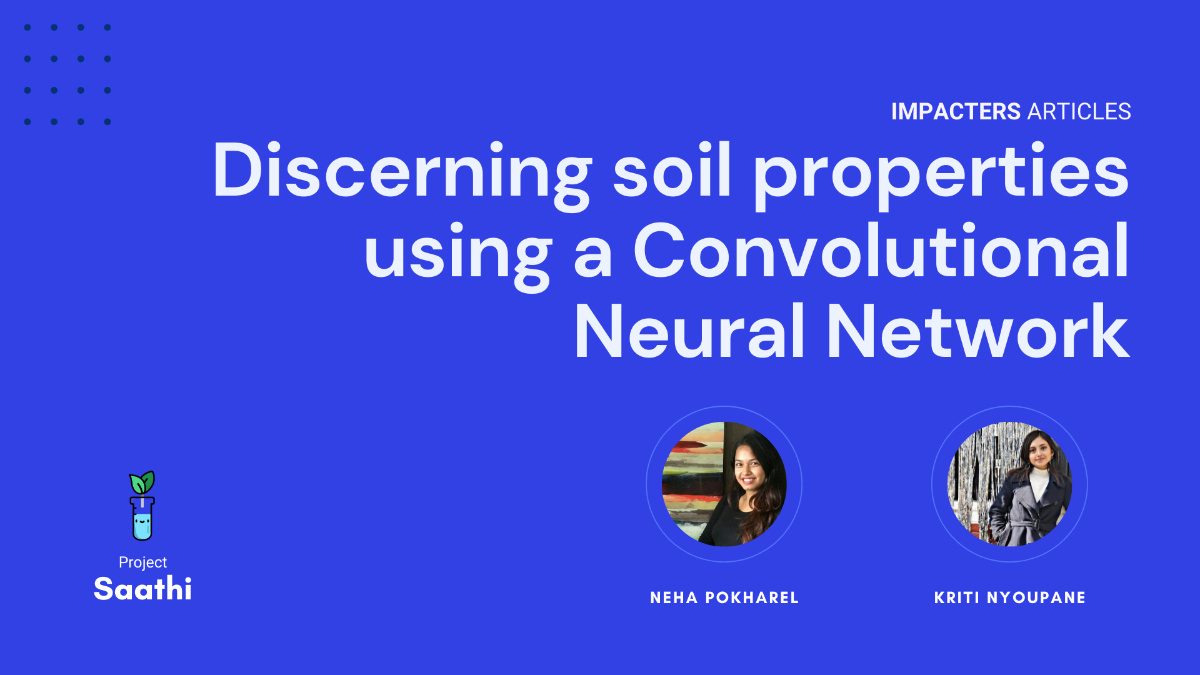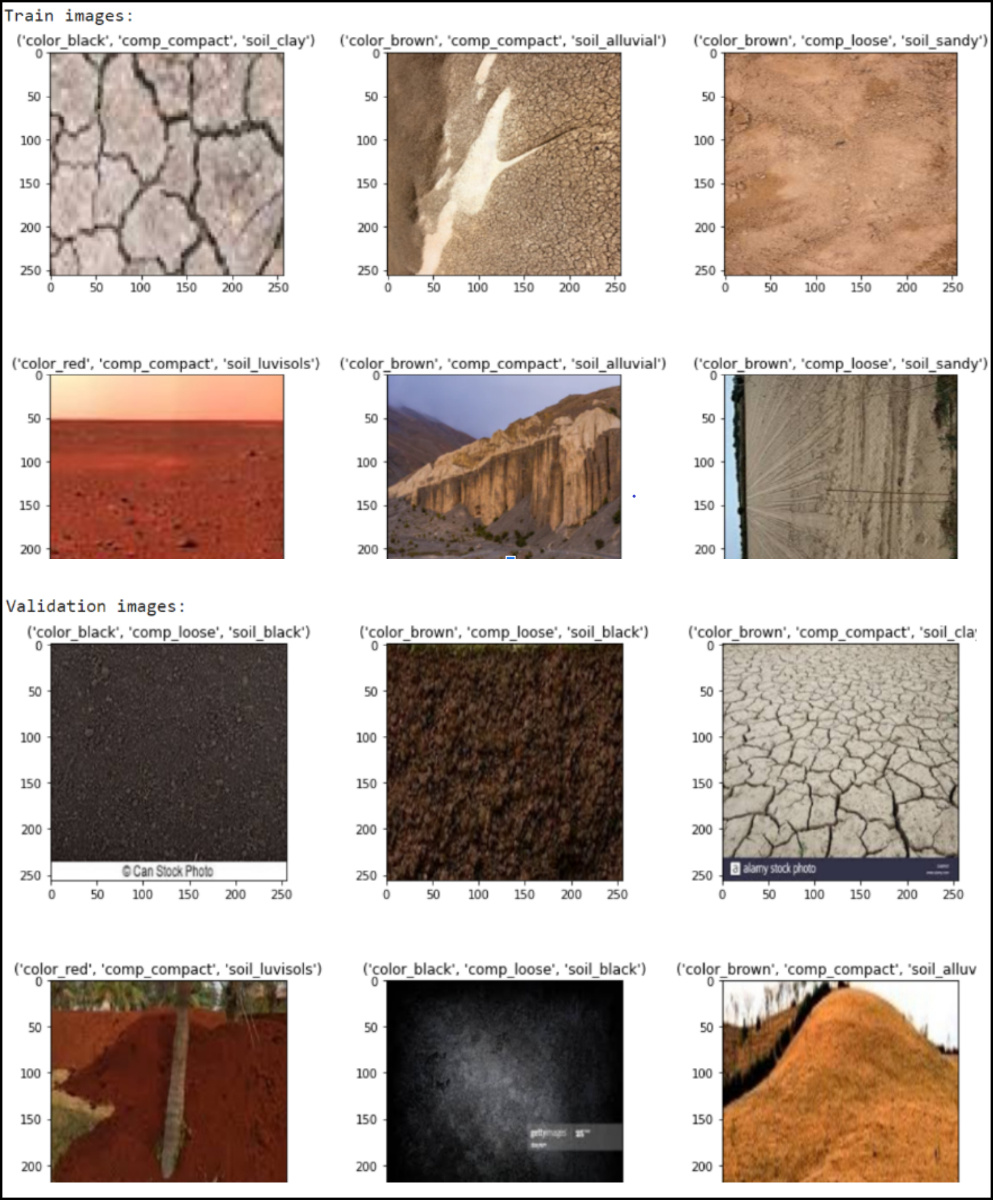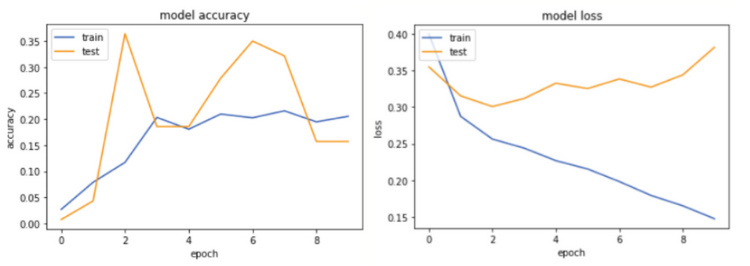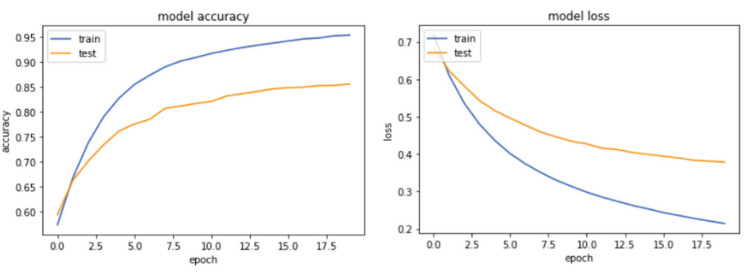# Discerning soil properties using a Convolutional Neural Network

Soil characteristics play a major role in determining what crops can grow in a particular acreage. Therefore in this article, we will attempt to delineate soil properties and categorize them based on their type, color and compactness.

Authors: Neha Pokharel, Kriti NyoupaneProject Saathi aims to provide Nepali farmers with scientific analysis of their farms using a combination of robust sensors and computer vision. Our group, the Futurists, particularly focuses on improving prediction of crops based on the soil properties. We are currently working on extracting elaborate parameters and hidden patterns of a particular acreage using image analysis of soil which is later coupled with sensor data to provide meaningful insights on the current state of the farm. In this particular article we will focus on the fundamentals of developing deep learning models - specifically the steps involved in creating image classifiers using the convolutional neural network (CNN) and using it to predict soil features.

Soil characteristics play a major role in determining what crops can grow in a particular acreage. Therefore in this article, we will attempt to delineate soil properties and categorize them based on their type, color and compactness. The following list consists of various soil textures, compactness and colors that are commonly found in Nepal, which we will use to train our model and ultimately make predictions.

``````# Defining classes
type_classes = [
"clay",
"loam",
"loamy_sand",
"sandy_loam",
"clayley_loam",
"loamy_clay",
"sandy",
"sandy_loam",
]
color_classes = ["brown", "black", "yellow", "red", "grey"]
compactness_classes = ["compact", "loose"]``````

The training dataset is labeled accordingly using the following folder structure:

``texture > color > compactness.jpg/compactness.jpeg``

However, before we begin, it is necessary to clarify the limitations of our dataset. Firstly we are using a simulated dataset and therefore the labels are not completely accurate. Secondly, our model is limited by the small size of the dataset. Typically a production scenario entails better and larger training data and a mix of various machine learning techniques, which are beyond the scope of this article.

1. Setting up

We will begin by importing some existing libraries and setting up our environment on Google Colab.

``````from keras.layers.convolutional import Convolution2D, MaxPooling2D
from keras.layers.core import Dense, Dropout, Activation, Flatten
from sklearn.preprocessing import MultiLabelBinarizer
from sklearn.model_selection import train_test_split
from keras.models import Sequential
import tensorflow.keras as keras
import matplotlib.pyplot as plt
from numpy import asarray
from PIL import Image
import pandas as pd
import numpy as np
import os
drive.mount("/content/gdrive") # our dataset is located on google drive, therefore we will mount the drive``````

The image dataset is loaded using our custom `load_soil_images` function. All images are first resized to a dimension of 256x256 pixels to ensure that our neural networks receive inputs of the same vector length. It is necessary to select a reasonable image dimension that is a good trade-off between quality and size. Selecting a small image dimension may result in higher loss of information pixels or image deformation. On the other hand, selecting big image dimensions may lead to bigger neural networks, with higher levels of complexity and resource requirements.

``````# Function for loading the dataset
x = []
y = []
soil_type = []
soil_color = []
soil_compactness = []
channel = 3
data_dir = '/content/gdrive/My Drive/Agriculture_Dataset'
for (soil, t) in enumerate(type_classes):
for (color, c) in enumerate(color_classes):
for (compact, o) in enumerate(compactness_classes):
image_path = data_dir + '/' + t + '/' + c + '/' + o
for file in os.listdir(image_path):
if file.endswith('.jpeg') or file.endswith('.jpg'):
img = Image.open(os.path.join(data_dir, t, c,
o, file))
(width, height) = img.size
newsize = (256, 256)
img = img.resize(newsize)
pixels = asarray(img)
pixels = pixels.astype('float32')
pixels /= 255.0
if pixels.shape == (256, 256, 3):
x.append(pixels)
z = ['soil_' + t, 'color_' + c, 'comp_' + o]
y.append(z)
return (x, y)``````

x and y denote normalized values of images and labels respectively.

Now that we have vectorized our image dataset and have respective labels, we can split the dataset into training and test datasets:

``X_train, X_test, y_train, y_test = train_test_split(x, y, test_size=0.2, shuffle=True, random_state=42)``

In our example, we will allocate 80% of the data for training and 20% for testing. Shuffle is set to true so that the order of the dataset is randomized before splitting. Additionally, setting an integer as a `random_state` ensures that the split is the same over multiple executions thus making debugging much easier.

2. Augmentation

Since the dataset is small, it may be beneficial to perform data augmentation. Various augmentation techniques such as flip (horizontal and vertical), rotate, skew, stretch etc. can be applied to create a bigger dataset. Following example demonstrates a vertical flip:

``````for i in range(len(X_train)):
X_train.append(np.flip(X_train[i], axis=0))
# axis = 0 represents flip is to be done on the vertical axis.
y_train.append(y_train[i])``````

3. Transforming and Verifying the data

The train and test variables are converted from list type to a high performance multidimensional numpy array of the same size.

``X_train=np.array(X_train)``

We are working with multiple labels that are in the string format. These are converted to numeric arrays using the `MultilabelBinarizer`.

``````mlb = MultiLabelBinarizer()
y_train= mlb.fit_transform(y_train)
Y_train.shape``````

`MultiLabelBinarizer` creates a binary matrix, where the columns correspond to the classes provided by `mlb.classes_`.

Here’s a visualization of training and validation images with their respective labels which can be used to verify the validity of the data:4. Creating our model

One of the most challenging tasks is determining a reasonable number of layers for our model. Fewer layers can lead to underfitting where the model fails to predict the data as it learns through least features. On the other hand, adding more layers could lead to overfitting where the model performs well on the training datasets but fails on new datasets.

Determining the number of layers is an iterative process and is unique to each scenario. For now we will follow the following methods to create a convolutional base model:

``model = Sequential()``

We can then add a common layer stack to our model that consists of a convolution layer and a max pooling layer. The convolution layer adds filters to the images (ex. sharpen edges), while the max pooling layer reduces the sizes of the images through downsampling.

``````model.add(Convolution2D(32,(3,3), activation='relu'))

The convolution layer also includes layers like dense layers and activation layers. The activation layer, especially, is used so that the network can learn complex patterns from the data.

``model.add(Dense(64, activation='sigmoid'))``

A sigmoid activation function is used in this example, which increases non-linearity in the output because of its logistic properties.

5. Model fitting

``````opt = keras.optimizers.Adam()
model.compile(
optimizer=opt,
loss=keras.losses.BinaryCrossentropy(from_logits=True),
metrics=[keras.metrics.BinaryAccuracy()],
)
history = model.fit(
X_train, y_train, epochs=25, validation_data=(X_test, y_test), shuffle=True
)``````

Once we have our model, hypertuning is used to adjust learning rate, optimizers, loss function, number of hidden layers etc. so that the model converges to better prediction accuracies and lower losses. In this example, an Adam optimizer is used which adjusts the learning rate and other parameters in every epoch and converges faster towards the minima.

Binary cross entropy, a loss function, compares each of the predicted probabilities to actual class output and calculates the score that penalizes the probabilities based on the distance from the expected value. Finally, we fit the model.

6. Results:Although we have successfully created our prediction model, as we can see from the results, the model accuracy is not adequate. We also see some overfitting because the validation loss increases while the training loss decreases. There are, however, a couple of steps we can implement to get better training accuracy. The first step would be to reduce simulated data and add a high number of real image datasets. Other steps include hyperparameter tuning that consists of adjusting values like dropout, learning rate etc.

The purpose of this article is to demonstrate primary steps of creating a machine learning model. Our group, however, extended this example with better training datasets, pre-trained MobileNet model, and numerous parameter tunings to reach accuracies of up to 85% on test datasets. The following figure shows training accuracy of 95% and test accuracy of 85%. The losses are iteratively converging to smaller values of around 0.37, which covers our use cases for project Saathi.